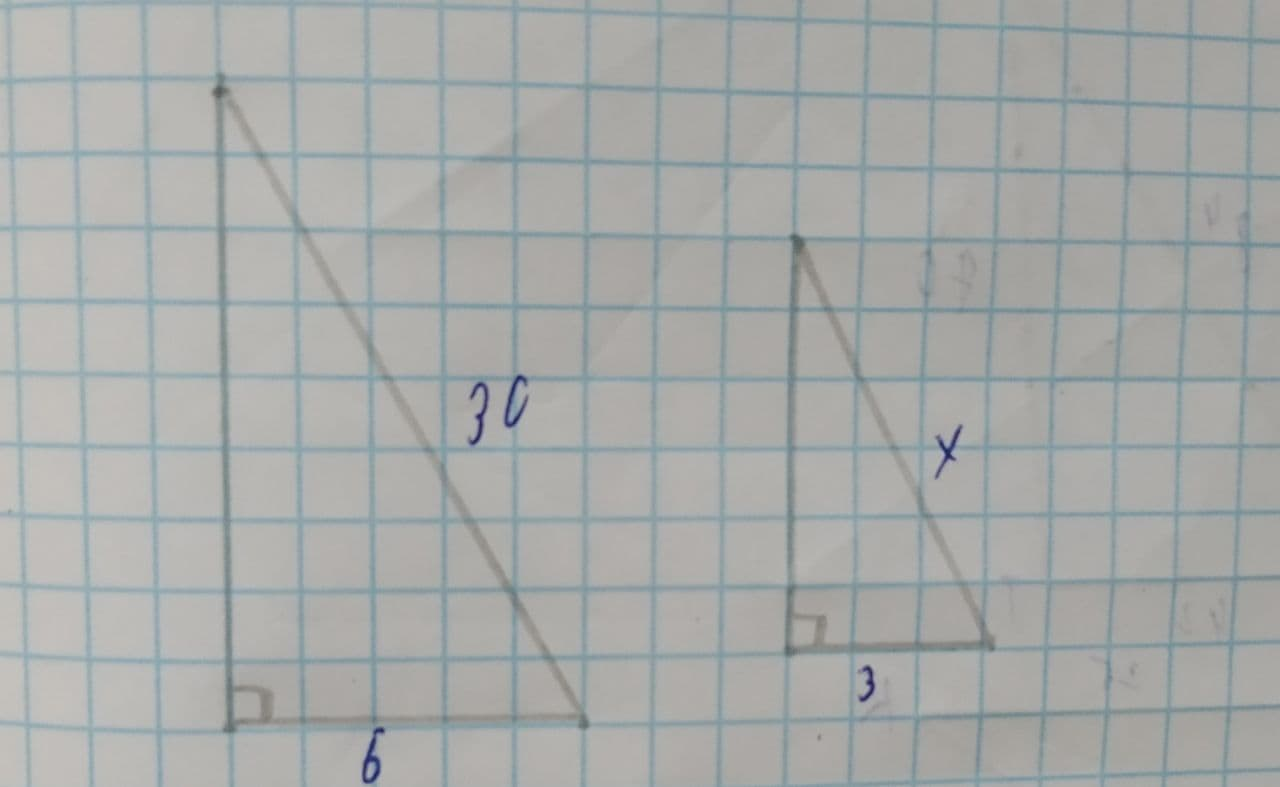# If the two triangles are similar by hypotenuse-leg similarity, what is the value of X?Carol Gates 2021-08-05 Answered
If the two triangles are similar by hypotenuse-leg similarity, what is the value of X?
Given,You can still ask an expert for help

• Questions are typically answered in as fast as 30 minutes

Solve your problem for the price of one coffee

• Math expert for every subject
• Pay only if we can solve itLatisha Oneil
Now,
Since, the two triangles are similar.
So, by using the hypotenuse−leg similarity, we have
$\frac{30}{6}=\frac{x}{3}$
$⇒5=\frac{x}{3}$
$⇒x=15$
$\therefore$ The value of x is 15.